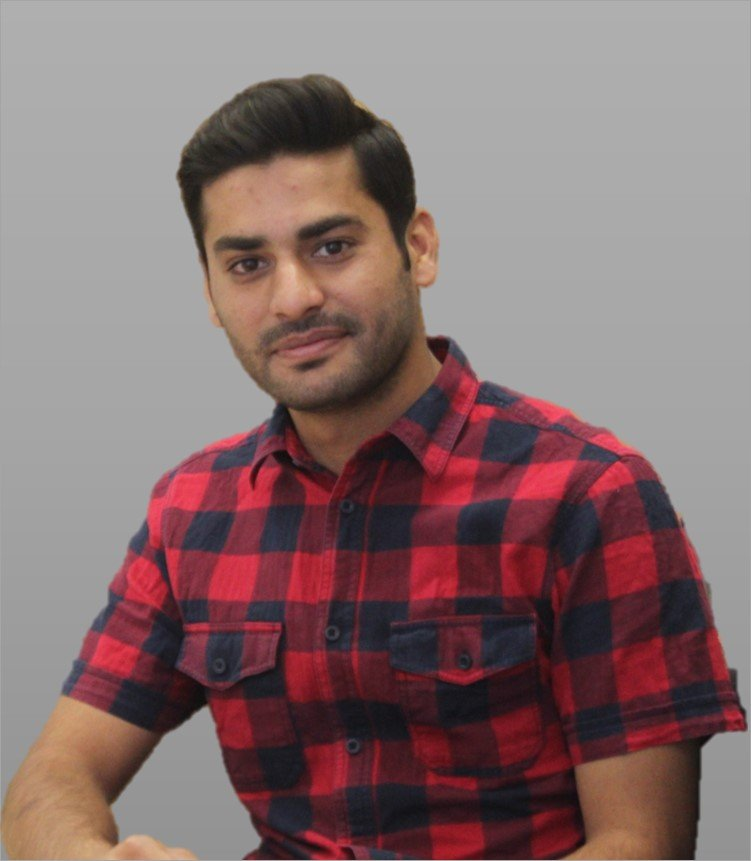# Numerical Mathematics

## Major research interests

• Convergence, stability and accuracy of numerical methods, for partial differential equations, analysis and development of numerical methods, finite element methods, finite volume methods, finite difference methods, dicontinuous Galerkin methods, kinetic schemes
• Theoretical and numerical study of systems of conservation and balance, especially in gas dynamics, multi phase mixtures, laser induced gas bubbles
• Riemann problems for systems of hyperbolic conservation laws, resonant waves, phase transitions
• Analytical and numerical methods for population balance equations in chemical and bio process engineering, existence and uniqueness of solutions, cell average method, finite volume schemes, discontinuous Galerkin methods

## Current PhD students in the IMPRS programWebsite
PhD project: Forced Periodic Non-isothermal Operation of Chromatographic Columns

## Jared Ouma Okiro

PhD thesis: Analytical and Numerical Investigation of an Intracellular Calcium Dynamics Model
(May 2, 2017)

## Carlos Cueto Camejo

PhD thesis: Biological population balance equations with non-local behavior and related Hamilton-Jacobi equations
(Jul. 10, 2013)

## Shumaila Javeed

PhD thesis: Numerical simulation of nonlinear chromatographic processes
(Jun. 25, 2013)

## Vincent Ssemaganda

PhD thesis: The Dynamics of the Becker-Döring System of Nucleation Theory applied to Process Engineering
(Sep. 26, 2011)

## Ankik Kumar Giri

PhD thesis: Mathematical Theory for the Dynamics of Coagulation-Fragmentation Equations for Process Engineering
(Oct. 25, 2010)

Go to Editor View